# Prism Spectrometer

\$650.00

Code Number: P0012

Brand: AELAB

#### Purpose:

• Understanding the structure and use of a prism spectrometer to observe typical spectral lines of He, Hg and Iodine.
• To determine the angle of the prism  A.
• To find the angle of minimum deviation and the refractive index of the material of the prism

- +

#### Prism spectrometer experiment including:

1. Student spectrometer

2. Power Supply for lamps

3. Spectra lamp: Mercury, Iodine, Helium

4. Prism

• Understanding the structure and use of a prism spectrometer to observe typical spectral lines of He, Hg and Iodine.
• To determine the angle of the prism  A.
• To find the angle of minimum deviation and the refractive index of the material of the prism

# Prism spectrometer experiment

#### I. Structure:

a) Collimator : It consists of a main tube at the one end of which there is a small draw tube fitted with a well corrected achromatic convergent lens with f.1,=178 mm, and the other end carries an adjustable slit. The draw tube can be moved helically inside the main tube by hand, in order to place the slit in the focal plane of the lens so as to give parallel rays. The collimator is mounted on a spring loaded support. The support is integral with the base.

b) Prism Table : The prism table is supported on a 175 mm. Diameter circular scale graduated in degrees. The circular scale is rotatable about the central axis and is provided with a clamping screw. The fully adjustable prism table with three leveling screws has screwed holes for fixing the prism and grating holders.

c) Telescope : The telescope is astronomical type consisting of main tube at one end of which there is draw tube fitted with an achromatic objective, and the other end carries a Ramsden's eye piece with cross wires. The draw tube can slide inside the main tube helically by hand. The telescope mounted on heavy bearings can be rotated about the 0 vertical central axis of the scale. Avernier reading to 0.1 degree is fixed to telescope arm. There is one clamping screw to fix the telescope and a tangent screw for slow motion of the telescope.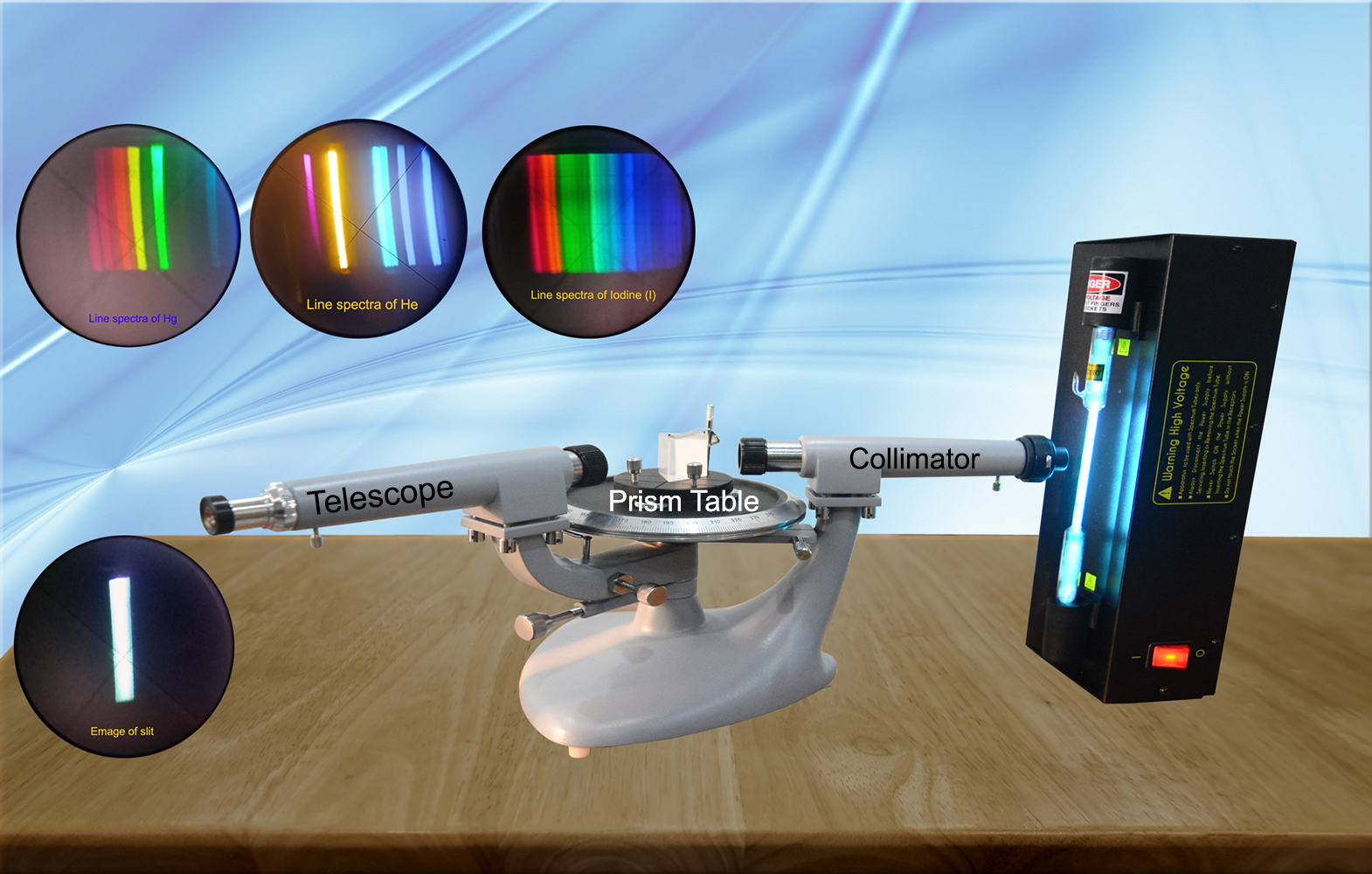Prism Spectrometer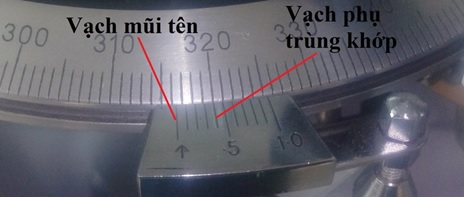Prism Spectrometer: Scale

## II. Preliminary Adjustment of spectrometer

First focus the cross wires by moving the eye piece in and out. This fixes the position of the eye piece, relative to the cross wires. Next pointing the telescope through an open window at some distant object lying at infinity such as an electric pole, adjust the distance between the eye piece and the objective with the help of helical arrangement, until there is no parallax between distinct image of distant object (at infinity) and the cross wire. When this is done the telescope must not be readjusted again during the experiment. It may happen that a second observer whose sight differs from that of the first is unable to focus the cross wires easily. He may readjust the eye piece provided that he does not alter any other part of the telescope.

The telescope is now turned towards the collimator with its slit illuminated with a monochromatic source of light. The collimator lens is then, adjusted with the help of helical arrangement until a distinct image of the slit falls on the cross wires and there is no parallax between the two. The instrument is now ready so that parallel rays pass from the collimator to the telescope

#### Expt. 1 : To determine the angle of the prism by any of the following two methods:

a) By turning the Telescope : Having adjusted the image symmetrical in the field of view of the telescope, on either face, allow the telescope to receive rays along AT1 from  face AC and along AT2 from the face AB each time the slit falling upon the across 2 wires. The Difference between the two readings (angle T1 AT2 ) is equal to twice the angle of prism i.e, 2A where A is angle of the prism. Half this difference gives the angles A.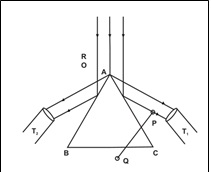b) By Turning the Table : Focus the telescope on the image of the slit by reflection from AB as shown. Note this reading. Keeping the telescope fixed rotate the prism table until the face AC is in position of AB, then AC has trough an angle i.e. the rays once again be reflected  in the orignal direction. The difference between the two reading is equal to θ i.e.  the angle in A  = (180  - θ).

#### Expt. 2: To find the angle of minimum deviation and the refractive index of the material of the prism: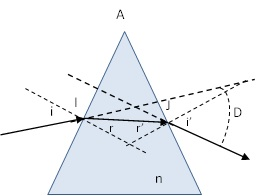#### The first, we concern the theory below:

At I: sini = n.sinr

At J: Sini’ =  n.sinr’

Angle of prism A = r + r’

Deviation Angle D = i + i’ -A

With angles are small enough:

i = n.r , i’ = n.r’ và A = r + r’

So that: D = (n-1).A

If Deviation Angle Dmin is munimum, then i = i’ = imin.

and r = r’ = A/2

So that Dmin = 2imin - A hay imin = (Dmin +A)/2.

So that: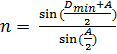(*)

#### Procedure:

+ Put the prism to rotation table. Adjust so that light comes out of the prism.

+ Rotate the telescope to catch a monochrome light (you can choose either yellow or green, or purple).

+ Gently rotate the prism table and telescope so that:

++ The image of the slit is always on the eyepiece.

++ The image of the slit moves in a direction that reduces the deviation angle (that is, the telescope always move anti-clockwise). If the image of the slit moves clockwise, then the more the angle of deviation increases, we must rotate it backwards.

++ Rotate the prism table (do not touch the prism) gently until the image of the slit can no longer advance counterclockwise, but starts moving clockwise then stops. Adjust so that the "+" sign is in the center of the image. That is the position to reach the minimum deviation angle Dmin.

++ Read the angle value on the scale φ1.

+ Remove the prism, rotate the telescope facing the collimator so that the center of the image of the slit is between the "+" sign. Reading angle φ0.

Angle of minimum deviation Dmin = φ1 - φ0

From Dmin, and Prism Angle A are measured above.

The refractive index of the material of the prism n is calculate by formula (*) above.

Do again with other monochrome light. Then conclude that:  The refractive index of the material depends on the wavelength of light.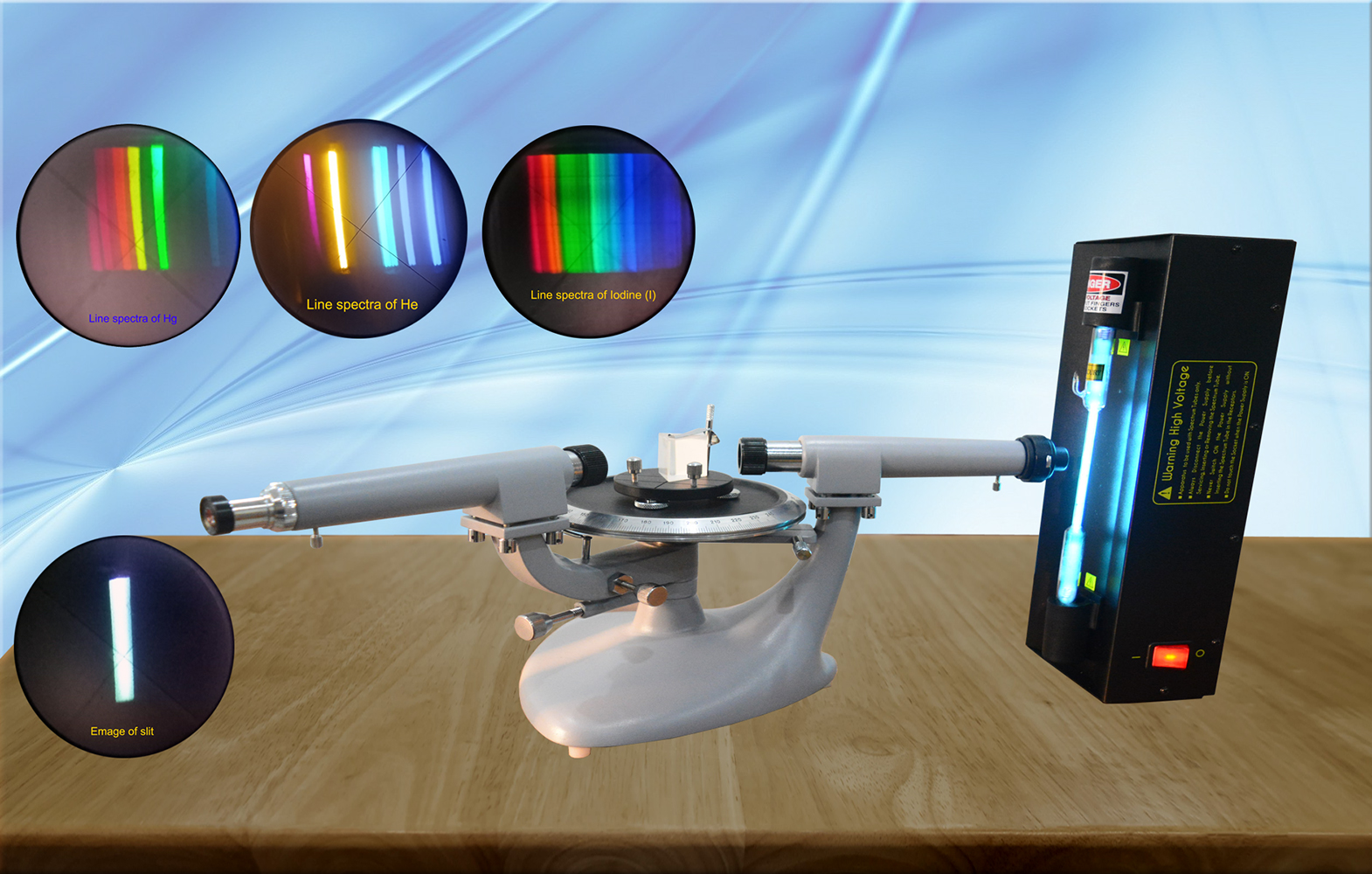Prism Spectrometer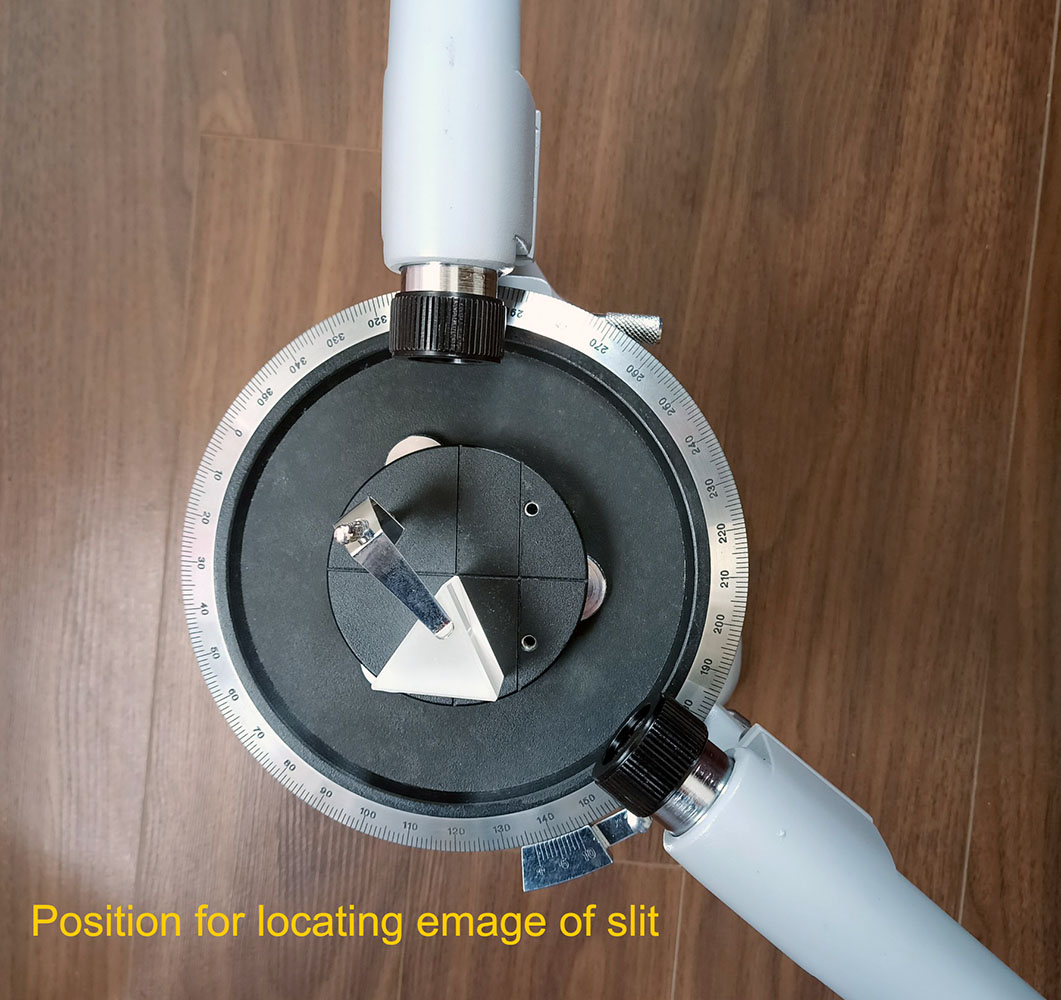Student Spectrometer: Prism table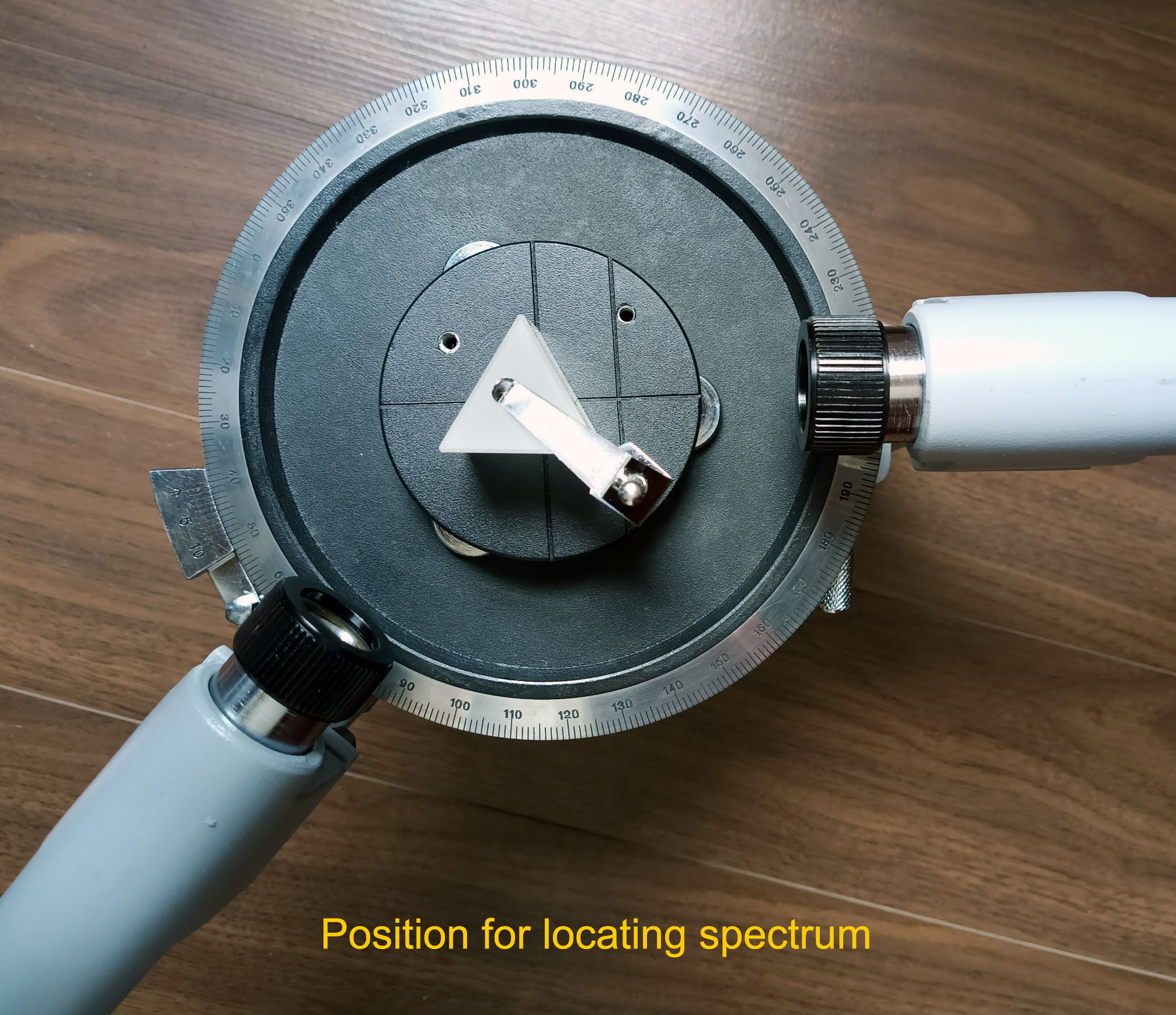Student Spectrometer: Prism table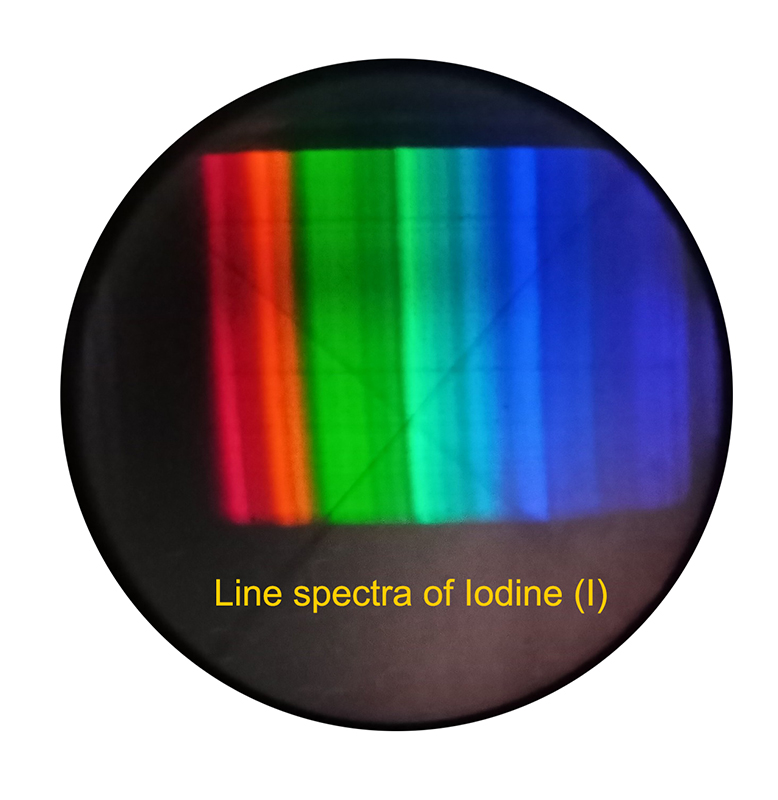Student Spectrometer: Spectra line of Iodine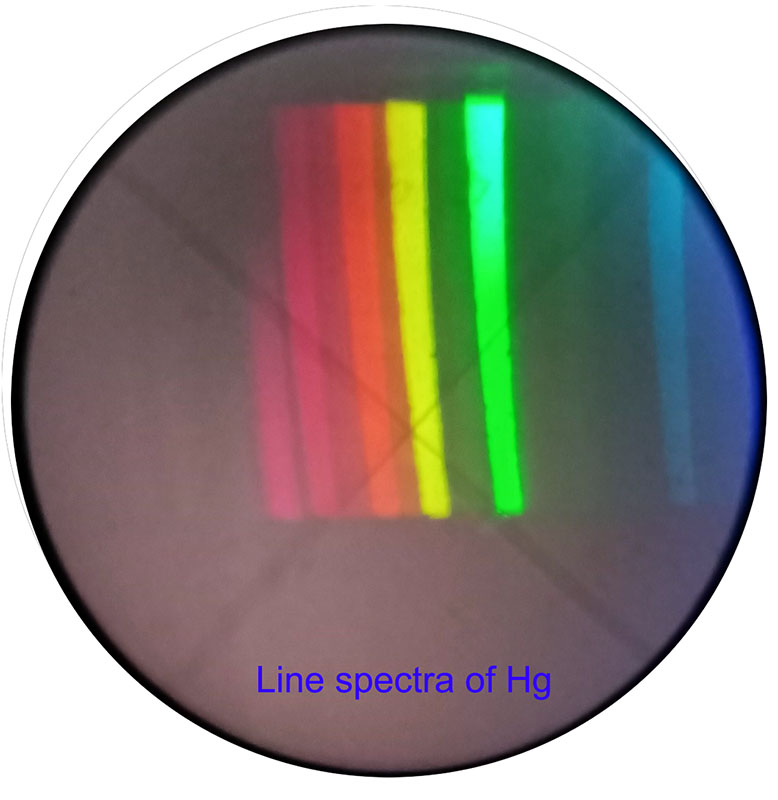Student Spectrometer: Spectra line of Mercury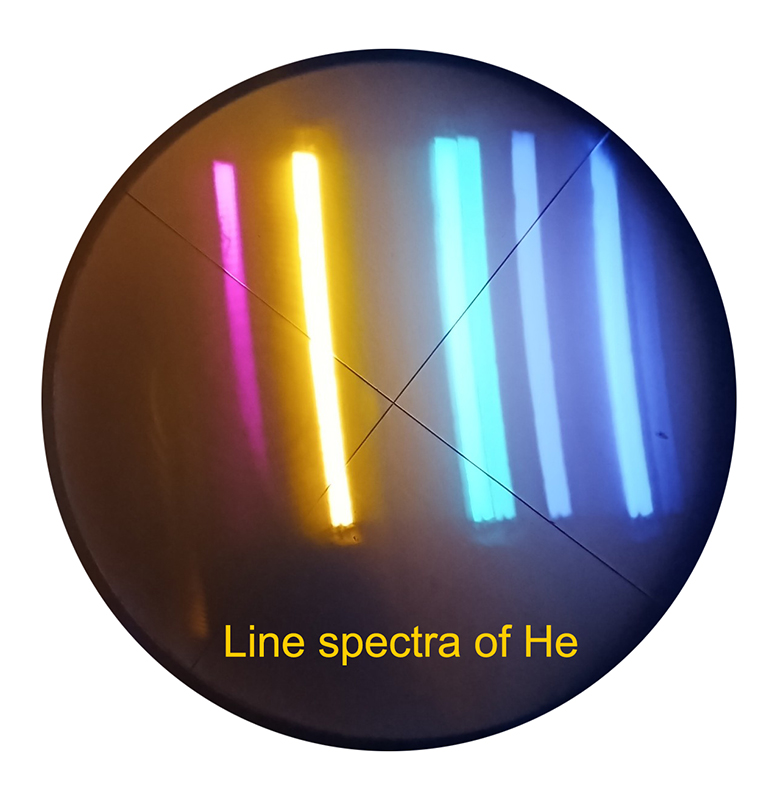Student Spectrometer: Spectra line of Helium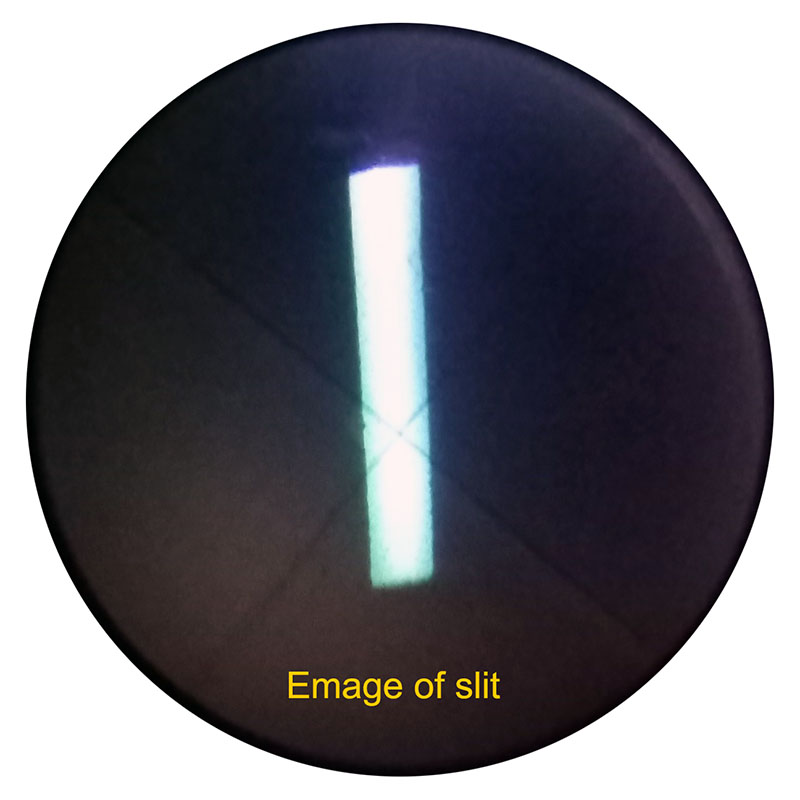Student Spectrometer: Slit